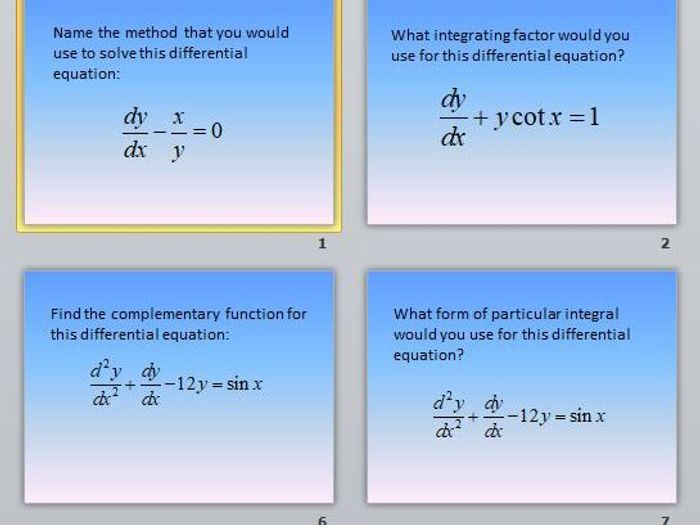This collection of resources provides everything you need to teach FP2 differential equations (first and second order), for the Edexcel syllabus. There is a 15-page booklet of notes and worked examples (full solutions provided). There are two Powerpoints quizzes, which can be used for revision or practice. A gap-fill sheet helps students become more familiar with the technical terminology used in first and second order differential equations. There is a sheet dedicated to substitutions in second order differential equations, focusing on finding the first and second derivatives for various substitutions (the part that students usually find the most difficult).
\$3.26
Save for later

Info

Created: Jan 30, 2018

Updated: Jul 12, 2019

Game, puzzle, quiz

pptx, 50 KB

First-Order-DEs-Quiz

Game, puzzle, quiz

pptx, 114 KB

Differential-Equations-Quiz

Activity

docx, 28 KB

Mathematical-Terminology-for-Differential-Equations

Report a problem